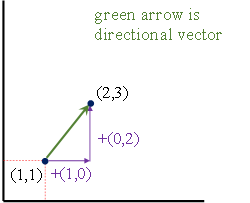top of page
Search
• Mohamed Salem

# The Real Reason Why the Gradient is the Direction of Steepest Ascent (and not descent)

Updated: May 30, 2022Machine Learning is currently an umbrella term for the set of clever mathematics that we use to build algorithms that can output decisions when fed only data. Very much like humans, algorithms built on data also need guidance while learning how to produce decisions. However, unlike the still enigmatic human learning process, algorithms are restricted in their “education” in the sense that they may only learn the way we program them to learn. Since most applications of machine learning algorithms are catered to a specific task (i.e. no generalizable learning required), we often resort to simple reward-penalty systems to guide our algorithms. These systems are embodied in what we call an objective function. An objective function is, loosely speaking, a mathematical relation that rewards the algorithm for making a right decision, and penalizes a wrong one.

Now that a system is in place, we have the tools to create algorithms that learn. However, we’re still asking the algorithm to make a single decision out of the set of all possible decisions, which could be a whole lot to handle, computationally. Enter the gradient. The gradient, simply described, is the slope (in our case, of the objective function) at a given point. It also has two excellent properties: (a) it considers all movement directions simultaneously, in the sense that if you have a 2-variable function, you don’t have to try all combinations of the first and second variable, rather the gradient considers both changes; and (b) the gradient is always the direction of steepest (read fastest) ascent. In the following paragraphs, we’ll show why the latter point always holds, and hopefully on the way, that will offer some elucidation regarding the former point.

I’ll begin this by defining what a vector is in the setting of this piece. We define a vector simply as an n-tuple of coordinates in an n-dimensional Euclidean space. In the provided example, we will be working in a two dimensional Euclidean space.The gradient is essentially the n-tuple holding each of the partial derivatives of some given function f with respect to each of its arguments (i.e. each of the dimensions that impact the function’s value) such that:

Note that this is simply just defining new vector (orange) on the gradient (red), by stretching and shrinking the coordinates of the gradient according to their counterparts in the directional vector:Now that all our definitions are in place, let’s examine the argument for the direction of the gradient being the direction of steepest ascent. Suppose we scale our directional vector to a unit vector (note, we can do this because scaling the vector doesn’t impact its direction), our formula then becomes:

But what is the direction of the gradient? In the previous figure, we seemed to know where the gradient arrow is pointing towards by treating each of the partial derivatives as coordinates in a direction vector. So, consider again the following example:

The derivative itself forces us to have a baseline movement in the positive direction by adding a positive quantity. This creates the direct relationship between the partial and the direction of increase. To prove that, I’m going to redefine the derivative as:Finally, one other way to show why this works is by going back to our previous formula:

Once again taking the magnitude of the directional vector to be 1 yields:

638 views
bottom of page